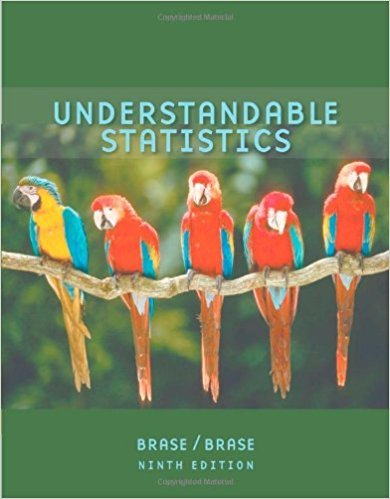×
Get Full Access to Understandable Statistics - 9 Edition - Chapter 9.1 - Problem 5
Get Full Access to Understandable Statistics - 9 Edition - Chapter 9.1 - Problem 5

×

# Veterinary Science: Colts The body weight of a healthyISBN: 9780618949922 213

## Solution for problem 5 Chapter 9.1

Understandable Statistics | 9th Edition

• Textbook Solutions
• 2901 Step-by-step solutions solved by professors and subject experts
• Get 24/7 help from StudySoup virtual teaching assistantsUnderstandable Statistics | 9th Edition

4 5 1 295 Reviews
30
3
Problem 5

Veterinary Science: Colts The body weight of a healthy 3-month-old colt should be about m 60 kg. (Source: The Merck Veterinary Manual, a standard reference manual used in most veterinary colleges.) (a) If you want to set up a statistical test to challenge the claim that m 60 kg, what would you use for the null hypothesis H0? (b) In Nevada, there are many herds of wild horses. Suppose you want to test the claim that the average weight of a wild Nevada colt (3 months old) is less than 60 kg. What would you use for the alternate hypothesis H1? (c) Suppose you want to test the claim that the average weight of such a wild colt is greater than 60 kg. What would you use for the alternate hypothesis? (d) Suppose you want to test the claim that the average weight of such a wild colt is different from 60 kg. What would you use for the alternate hypothesis? (e) For each of the tests in parts (b), (c), and (d), would the area corresponding to the P-value be on the left, on the right, or on both sides of the mean? Explain your answer in each case.

Step-by-Step Solution:
Step 1 of 3

Important Terms Observational Study­ Data gathered by only witnessing individuals without guiding or stating an input on them. Designed Experiment­ Data gathered by an examiner on individuals then runs trials each to test one or more variables. Cofounding Variables­ existing variables within the student that affect the response Randomization­ randomly assigned themes to experiments Theoretical Method­ Divide the number of results in the experiment taken by total number results Empirical Method­ Calculating the relative frequency Relative Frequency­ X/n X­ number of successes n­ number of results Response and Explanatory Variables X= Response Variable = dependent Y= Explanatory Variable­ Independent Goal X is what causes the change in Y Probability

Step 2 of 3

Step 3 of 3

##### ISBN: 9780618949922

Unlock Textbook Solution